# Om Prakash

•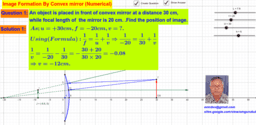### Method to solve Numerical based on reflection by convex mirror

Activity

Om Prakash

•### Numerical Problem based on 'Reflection by Concave Mirror'

Activity

Om Prakash

•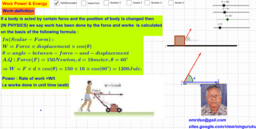### Definition & Explanation of Work & Power with the help of Numerical

Activity

Om Prakash

•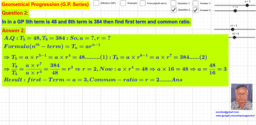### Geometrical Progression (GP Series) Explanation & Demonstration

Activity

Om Prakash

•### Use of delta-star combination in solving complicated resistance circuit

Activity

Om Prakash

•### Important Numerical of refraction by Curved refractive Surface

Activity

Om Prakash

•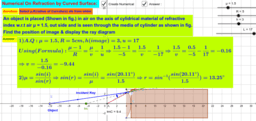### Numerical on refraction by Curved Surface

Activity

Om Prakash

•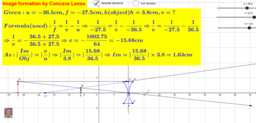### Numerical Solving of Concave Lense

Activity

Om Prakash

•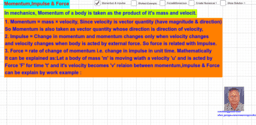### Explaination & Relation between Momentum. Impulse & Force

Activity

Om Prakash

•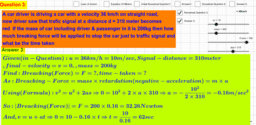### Numerical Solving based on Motion on Linear Path (Using Newton's Equation of Motion)

Activity

Om Prakash

•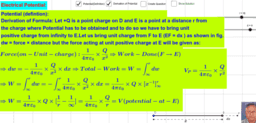### Derivation of Formula For Electrical Potential due to point charge

Activity

Om Prakash

•### Orbital Velocity,Time Period calculation of a satelite

Activity

Om Prakash

•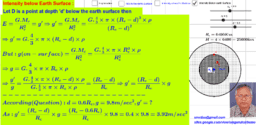### Gravitational Field Intensity due to Earth's Field at different Point and relation between g and G,

Activity

Om Prakash

•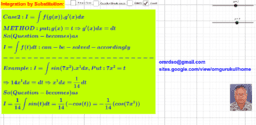### Integration By Substitution

Activity

Om Prakash

•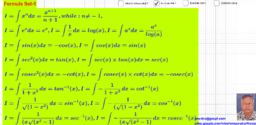### Integration (Introduction & Formula Display)

Activity

Om Prakash

•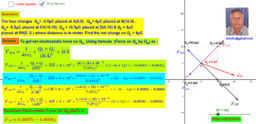### To find net electrostatic force on a given charge due to four charges placed at four different position

Activity

Om Prakash

•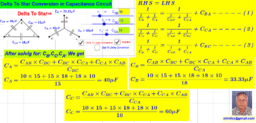### Formula derivation for Delta to Star to Delta Conversion in capacitance circuit

Activity

Om Prakash

•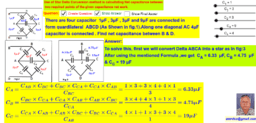### Numerical using STAR-DELTA Method to find net capacitance .

Activity

Om Prakash

•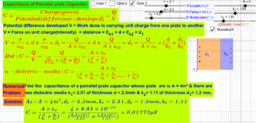### Derivation of capacitance of parallel plate capacitance

Activity

Om Prakash

•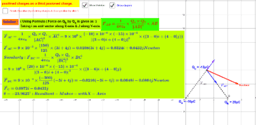### Numerical: Find out Electrostatic Force on a charge due to charges placed as decided

Activity

Om Prakash

•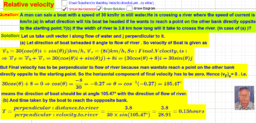### Relative Velocity Numerical #2

Activity

Om Prakash

•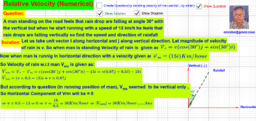### Relative Velocity Numerical #1

Activity

Om Prakash

•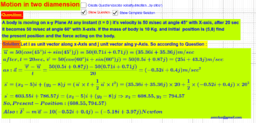### Relative Velocity (Numerical -3)

Activity

Om Prakash

•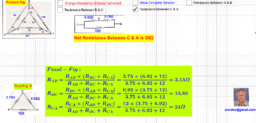Activity

Om Prakash